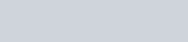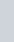Mark the correct alternative in each of the following:
Question:

Mark the correct alternative in each of the following:

If a digit is chosen at random from the digit 1, 2, 3, 4, 5, 6, 7, 8, 9, then the probability that it is odd, is

(a) $\frac{4}{9}$

(b) $\frac{5}{9}$

(c) $\frac{1}{9}$

(d) $\frac{2}{3}$

Solution:

GIVEN: digits are chosen from 1, 2, 3 4, 5, 6, 7, 8, 9 are placed in a box and mixed thoroughly. One digit is picked at random.

TO FIND: Probability of getting an odd digit

Total number of digits is 9

Digit that are odd number are 1,3,5,7,9

Total number of odd digits is 5

We know that PROBABILITY =Hence probability of getting an odd digit isHence optionis correct